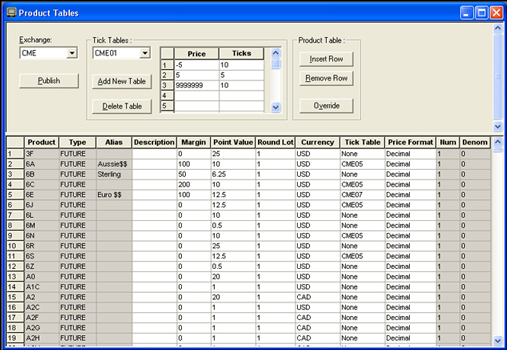← Back to X_TRADER® Help Library

### Guardian Documentation

#### Tick Size Calculation

The calculation of a product’s tick size requires data from both the Tick Table Setup and the Product Table Setup.To manually calculate tick size:

1. Calculate the base tick value by dividing the Product’s numerator by the denominator.
2. Refer to the associated tick table, and reference the correct upper price limit and Ticks multiplier.
3. Calculate the tick size by multiplying the base tick value by the tick table Ticks multiplier.

For example, the base tick value of a product may be listed as 1/100=.01, and the multiplier for all prices is 1.

Then the tick size for that product would be .01*1=.01